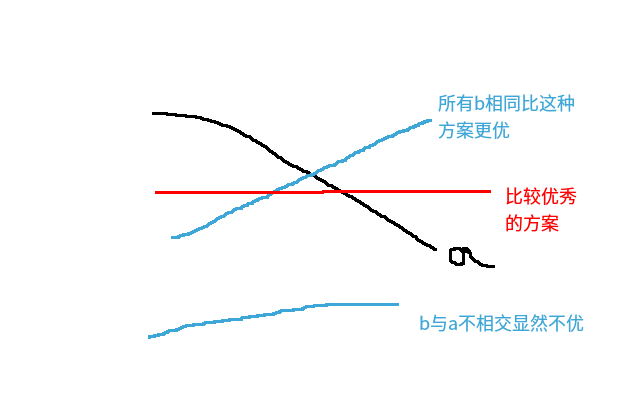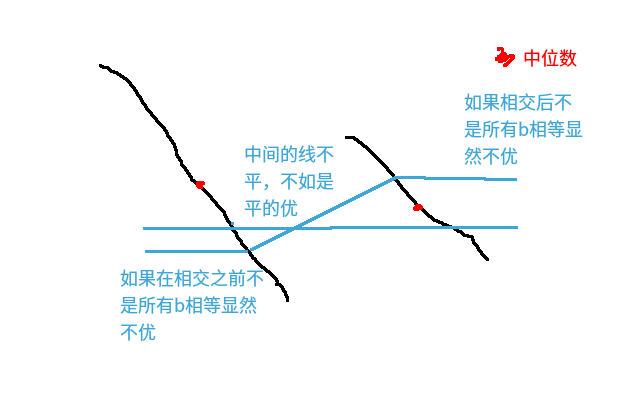#include<bits/stdc++.h>
using namespace std;
#define RI register int
int q=0;char ch=' ';
while(ch<'0'||ch>'9') ch=getchar();
while(ch>='0'&&ch<='9') q=q*10+ch-'0',ch=getchar();
return q;
}
typedef long long LL;
const int N=1000005;
int rt[N],sz[N],L[N],R[N],A[N],B[N];
struct node{int ls,rs,d,v;}t[N];
int n,js;LL ans;
int merge(int a,int b) {
if(!a||!b) return a|b;
if(t[a].v<t[b].v) swap(a,b);
t[a].rs=merge(t[a].rs,b);
if(t[t[a].rs].d>t[t[a].ls].d) swap(t[a].ls,t[a].rs);
t[a].d=t[t[a].rs].d+1;
return a;
}
int main()
{
for(RI i=1;i<=n;++i) {
++js,rt[js]=i,sz[js]=1,L[js]=i,R[js]=i,t[i].v=A[i];
while(js>1&&t[rt[js-1]].v>t[rt[js]].v) {
sz[js-1]+=sz[js],R[js-1]=R[js];
rt[js-1]=merge(rt[js-1],rt[js]),--js;
while(sz[js]>(R[js]-L[js]+2)/2)
rt[js]=merge(t[rt[js]].ls,t[rt[js]].rs),--sz[js];
}
}
for(RI i=1;i<=js;++i)
for(RI j=L[i];j<=R[i];++j) B[j]=t[rt[i]].v;
for(RI i=1;i<=n;++i) ans+=1LL*abs(A[i]-B[i]);
printf("%lld\n",ans);
return 0;
}


### 1 条评论

#### 【题解】 K凹凸序列 可并堆 BZOJ – 2171 – K-XZY · 2018年12月14日 10:22 上午

[…] $k = 2$，同 bzoj1367 sequence，只不过把问题中的上升改成不降（以及不升）[…]